# motion solution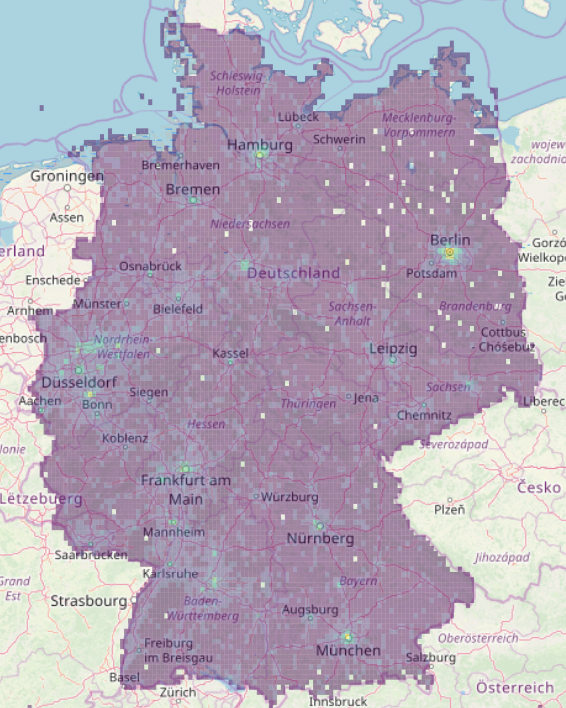density of gps events across germany

# data type

We get for each user a line with x and y coordinates, device type, accuracy in meters and time zone. For each coordinate pair we create an octree index for the given accuracy

## positions

ts id type x y accuracy tz octree n
1556146040 00002af79399fef8e… 0 52.2833 7.93245 17 7200 314223124321441 1

## densities

From the positions we calculate the densities grouping by octrees and we remove one octree digit every time the number of events is smaller than a threshold.

In between we calculate the average position and time for each box.

octree n x y ts
422331313214 35 7.5405 47.712 1554083921

We can than convert the octrees in polygons and display the density values with a color map rendering first the large boxes.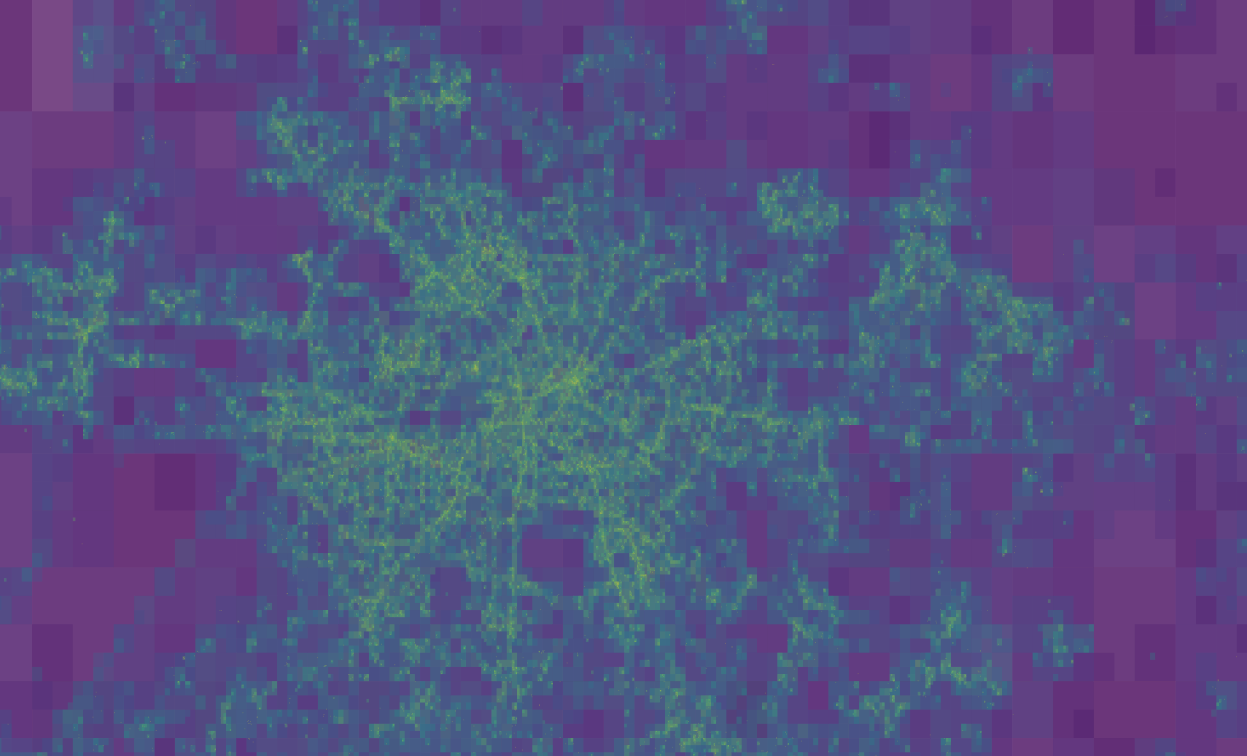densities in the city

We can clearly distinguish users driving on main roads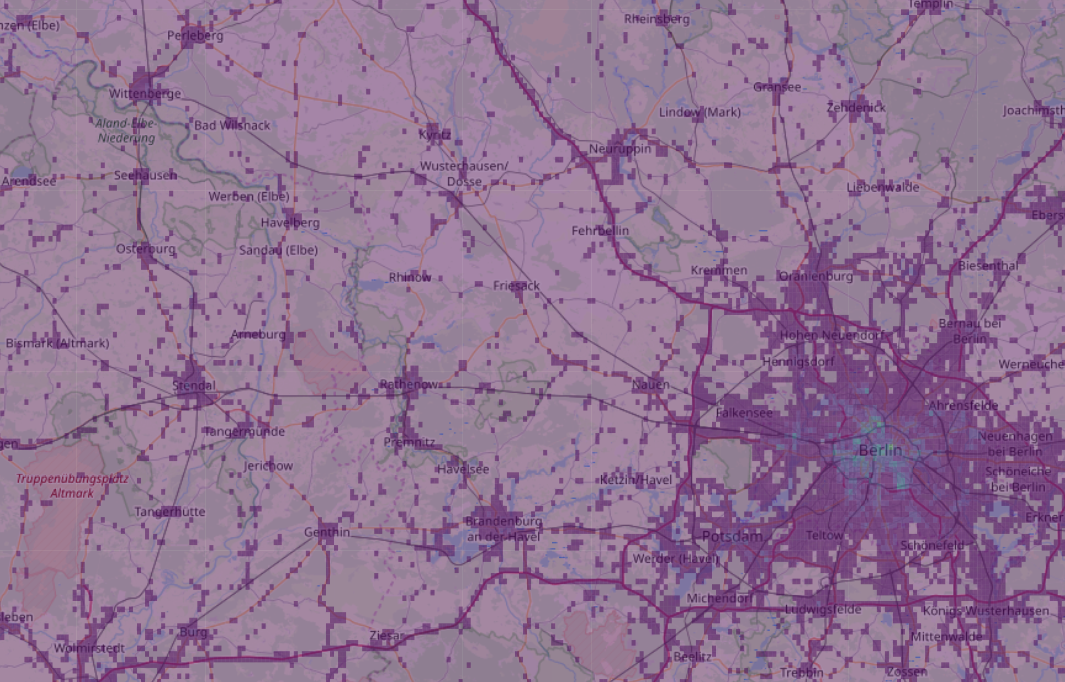density on the streets

# trajectories

We pivot all entries by the user id and we create a new dataframe where on each line we have id, time-space matrix, n trajectory points, bounding box in octrees, time interval

id tx n bbox dt
933a58bfed63df92570… [[1556210261, 13.497, 52.395], [155626... 976 423 15562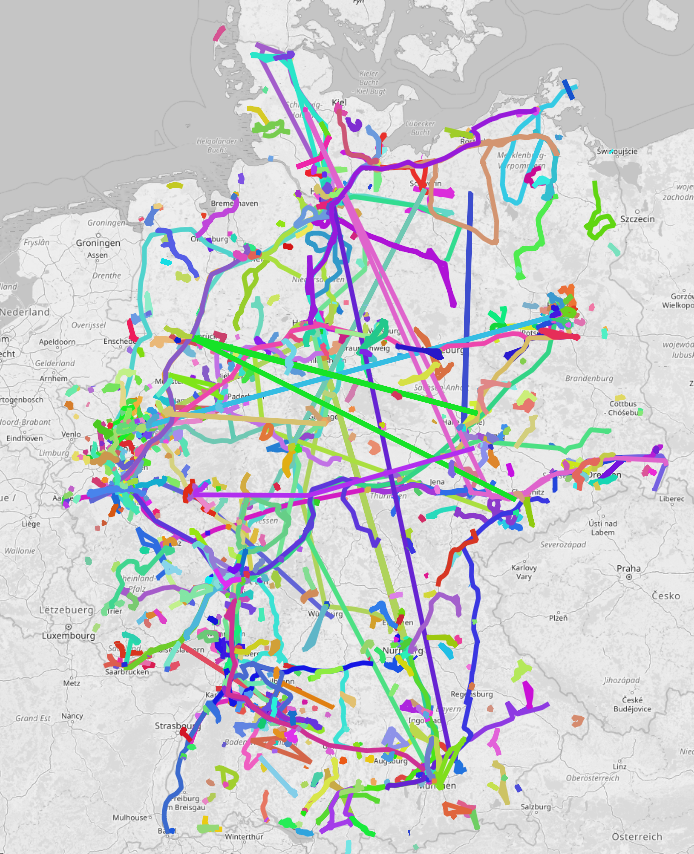trajectory country

All trajectories are defined by a bounding box which makes spatial filtering efficientdensities and trajectories

## frequencies

We create a dataframe each day to know how many users we see in the country

id ts
00002af79399fef8eb17522aedba3cedc45bb3cd193a34… 85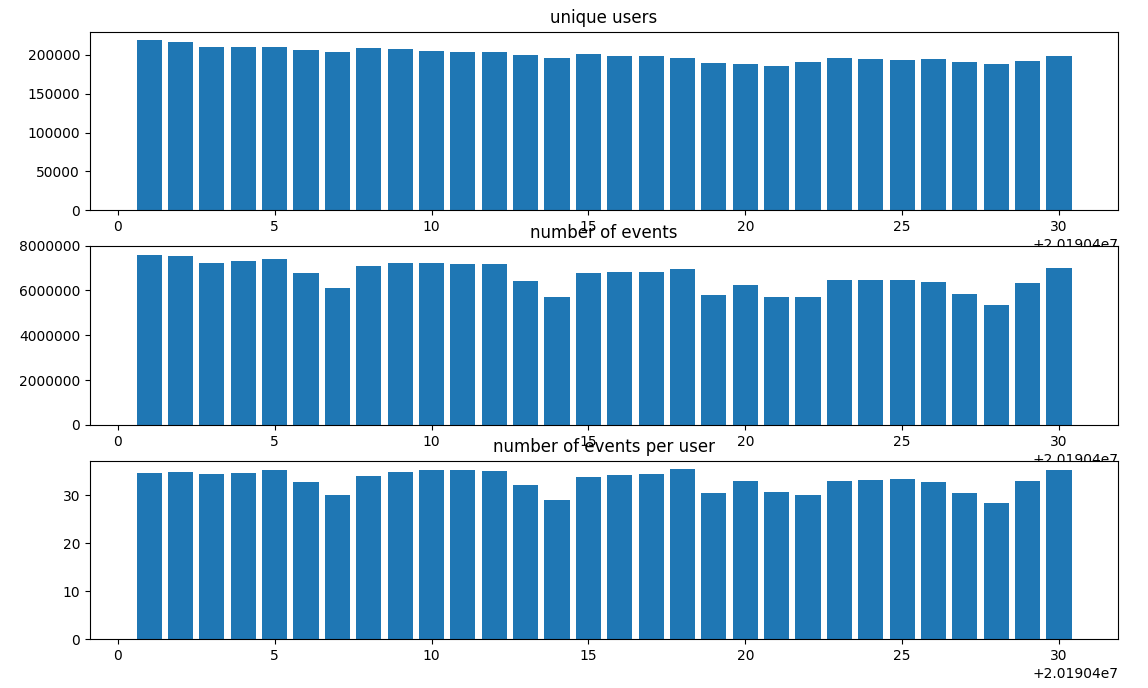user frequency

If we look at the distribution of events per users we notice extreme numbers and the median drops to 19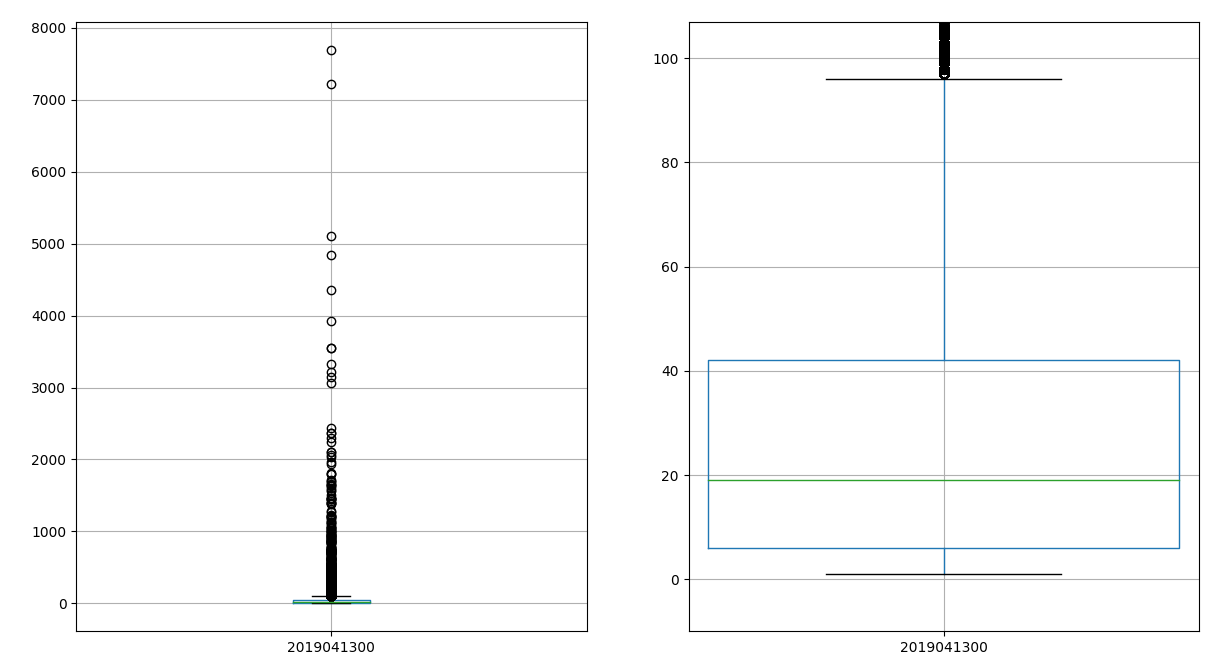user distribution

# Speed profile

We iterate over all trajectories and calculate the point to point space-time difference. We derive than the speed, the angle and the chirality of the segment.

octree n speed angle chirality
423144344341423 1.0 0.000008 0.957055 1.0

Where we calculate the speed as a normal equation of motion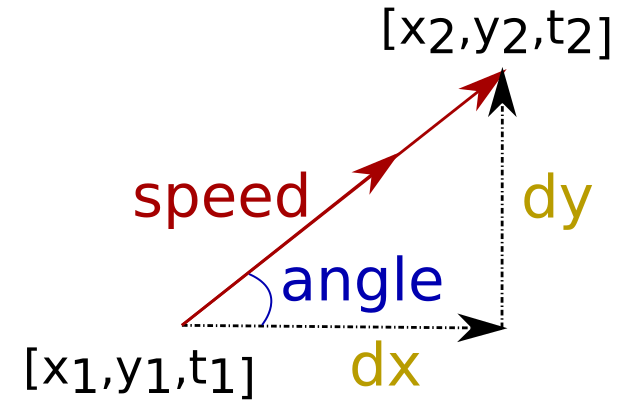speed calculation

To calculate the segment speed we need first to smooth the input variables inducing correlation between the points. Without smoothing data are too noisy to be processedspeed, no smoothing

If we use a running average space-time and their derivatives are better defined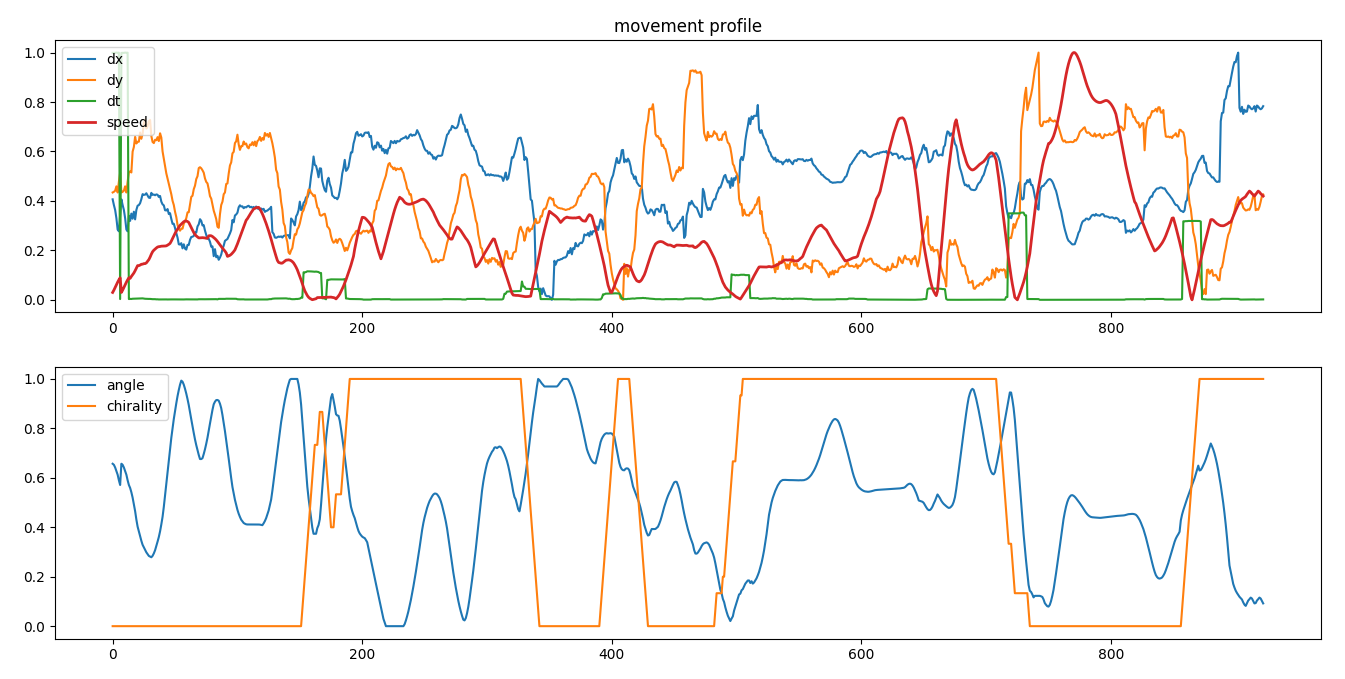speed, running average

If we use a Kaiser smoothing we have smooth profiles across all variables.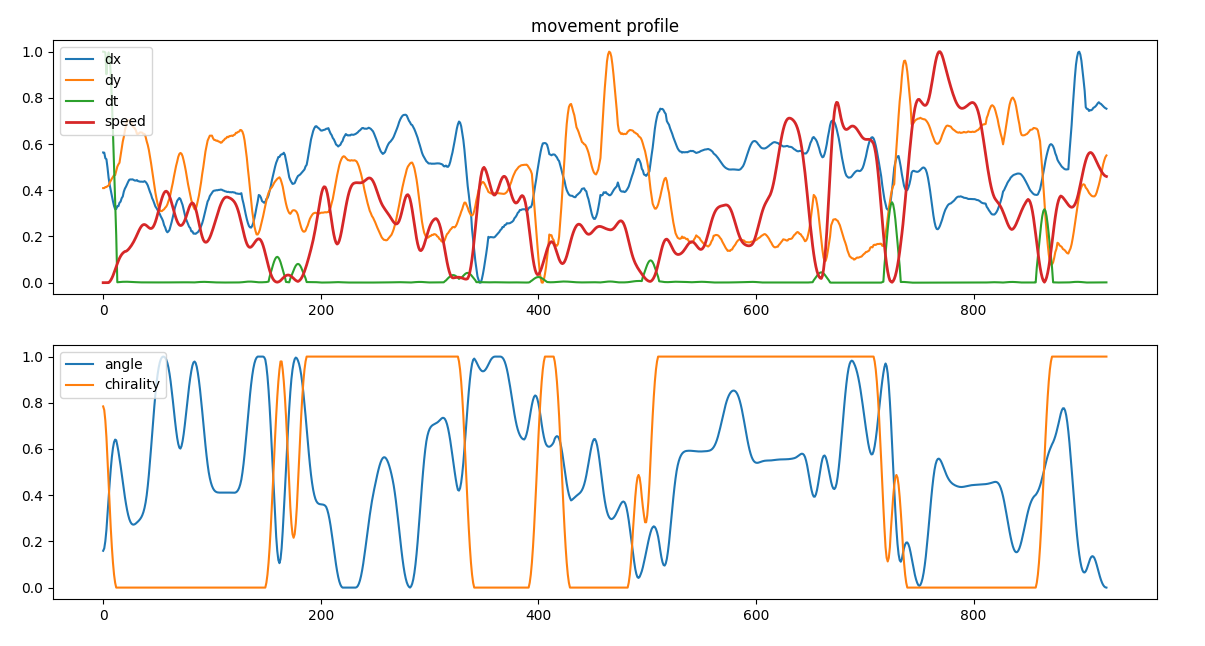speed, kaiser smoothing

In this way we can calculate the speed density across the city and clearly see the network infrastructure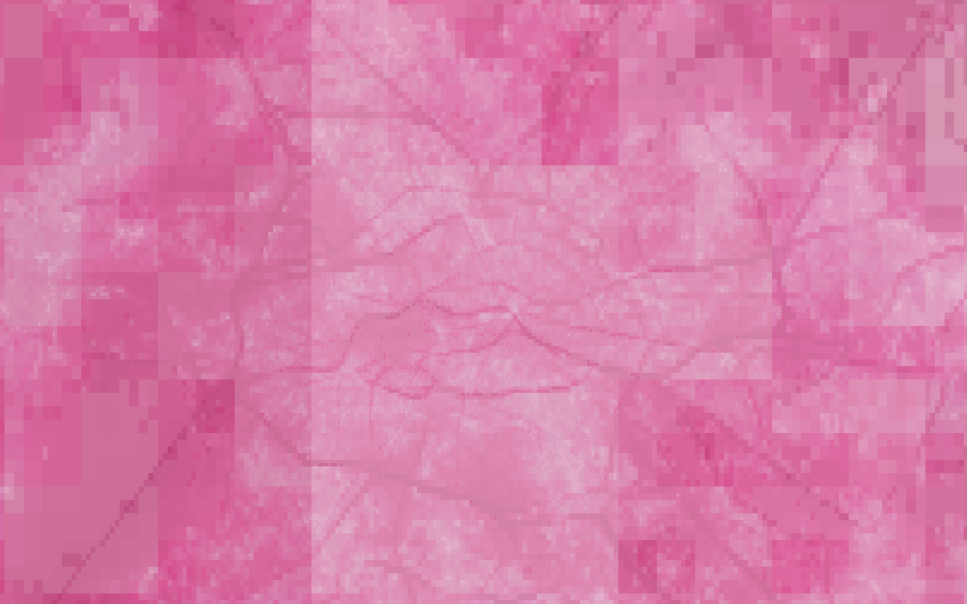speed density

We can also observe the distribution of chirality across the city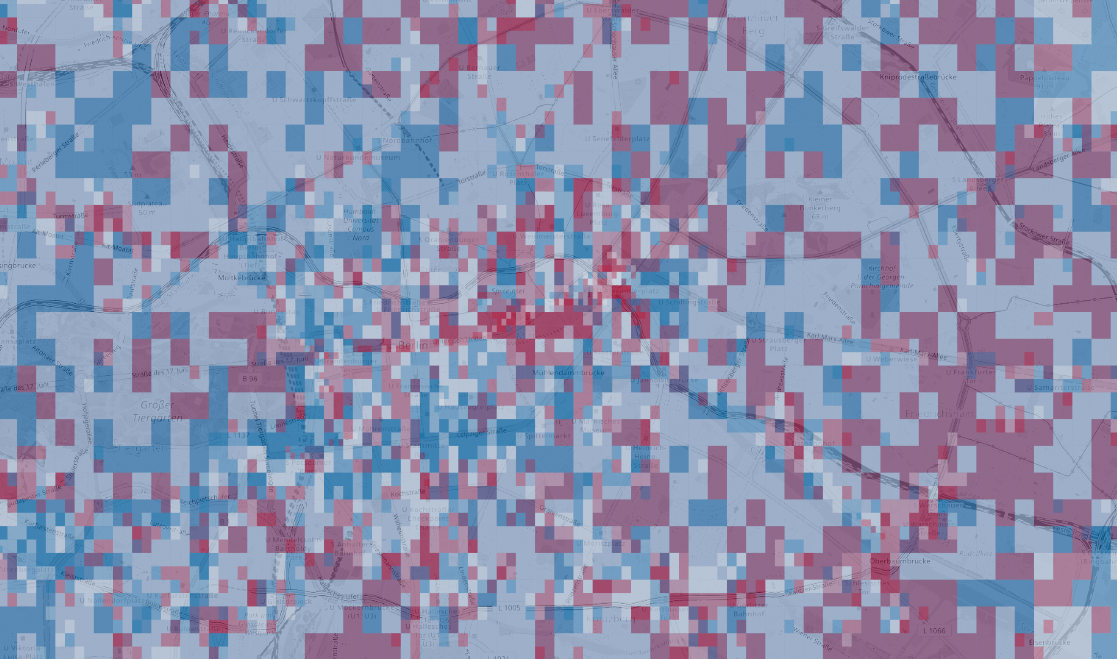chirality density

and the distribution of angles, interesting there are some spots strongly polarized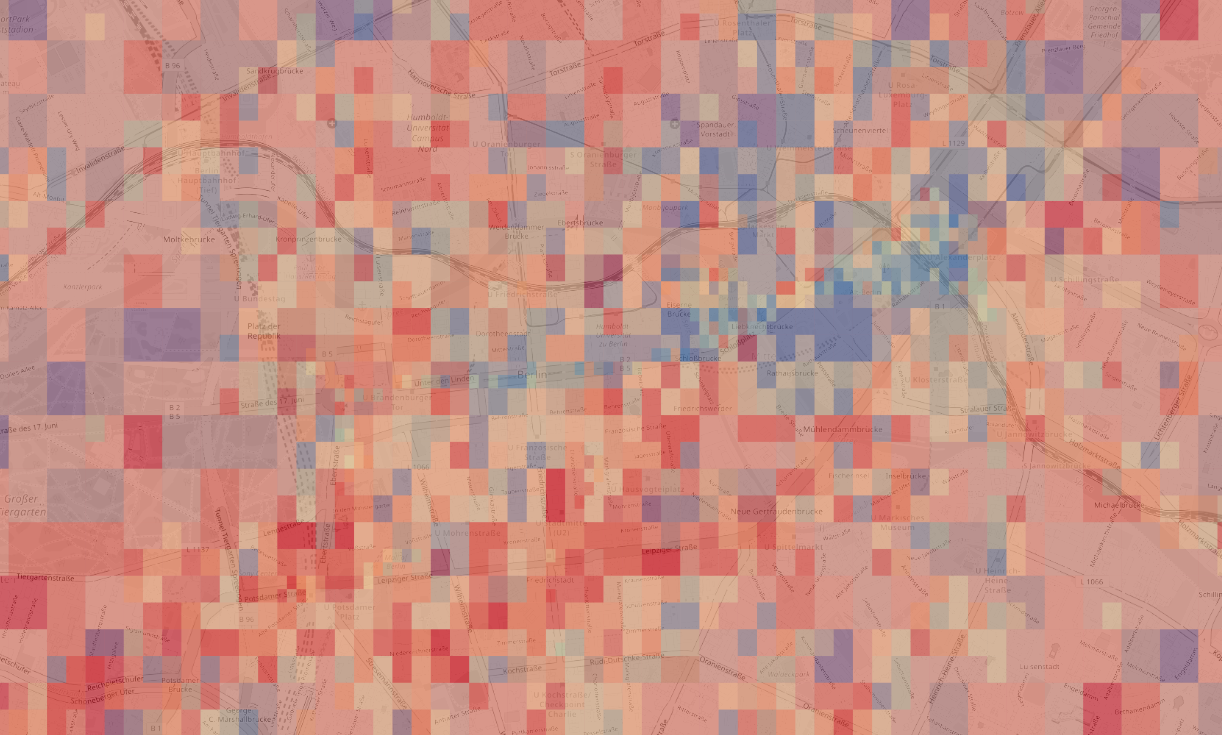angle density

## motion

If we set a speed threshold depending on the particular use case (long/short trips) we can divide motion from dwelling. We cluster the speed profiles when a velocity cross a threshold value. Below the threshold we have a dwelling, above a movement.

mean values

m_dt m_x m_y m_speed m_angle m_chirality m_m
4165 13.448 52.397 1.31E-06 1.33 0.5 1.80E-07 1248.57

standard deviations

s_dt s_x s_y s_speed s_angle s_chirality s_m
0.00026 2.58E-05 2.62E-06 0.553 0.234 0

first and last signal

x1 y1 t1 x2 y2 t2 sr
13.44 52.3 2285.5 13.44 52.397 5196.57 0.000143

User defined definition of activities and tripsspeed profile and the threshold defining activities

We can display the dwelling and the trips across the city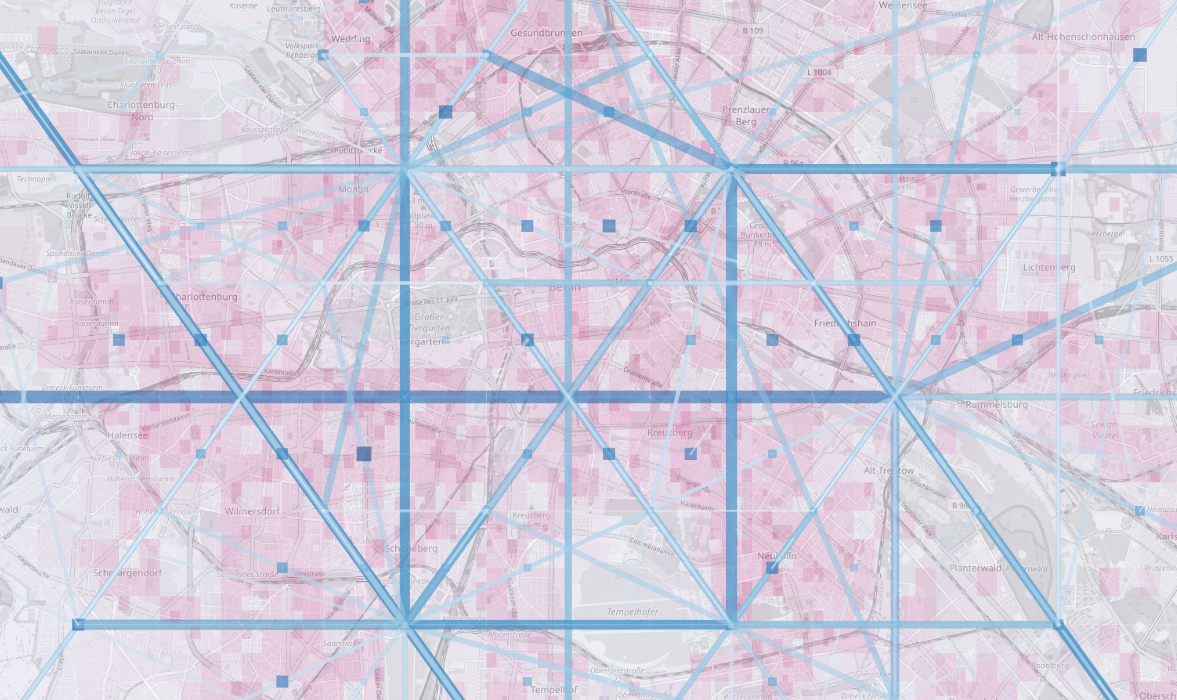dwelling and moving across the city

Changing the threshold we can distinguish different types of movemens and we have completely different figures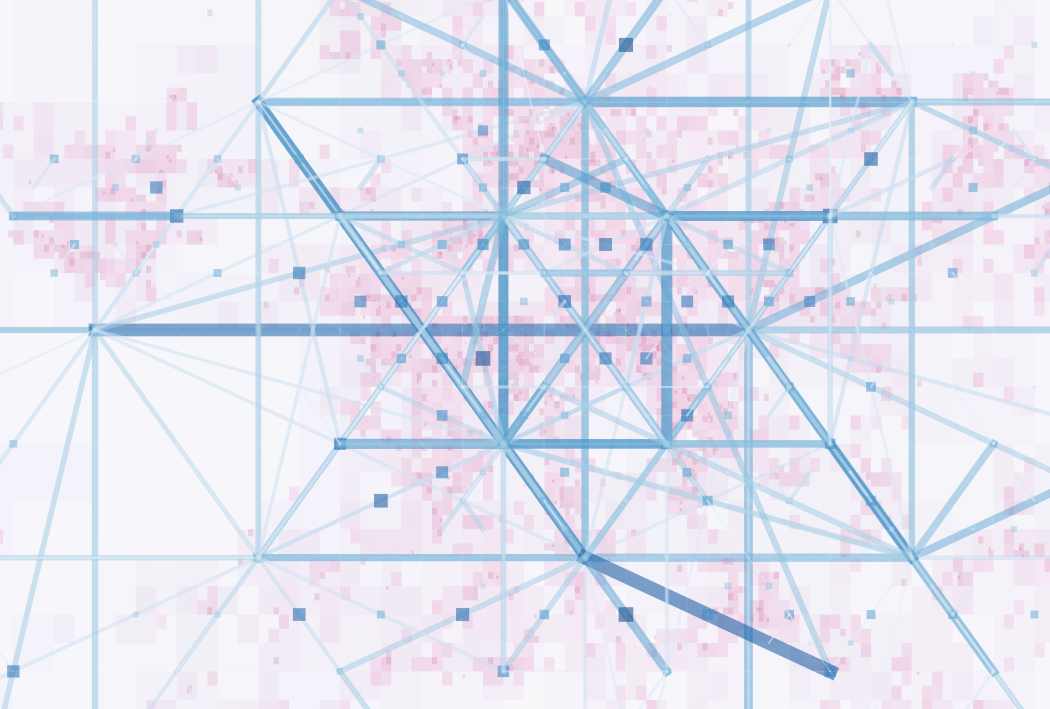moving and dwelling

We sum up relations grouping first on destinations and than on origins and iterate coarsing the geometry until we reach similar counts per leg.

We have many relations for each node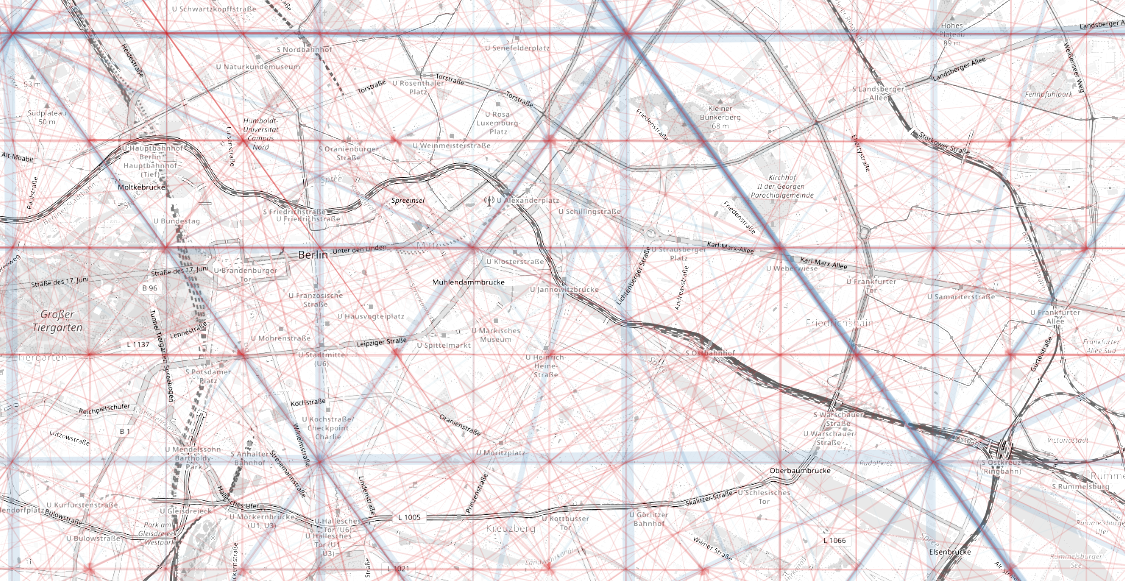inner city relations

## dwelling

Dwelling can be disconnected from the octree indexdwelling heatmap

# Retail

We want to compare mobile antenna connections with gps signal density,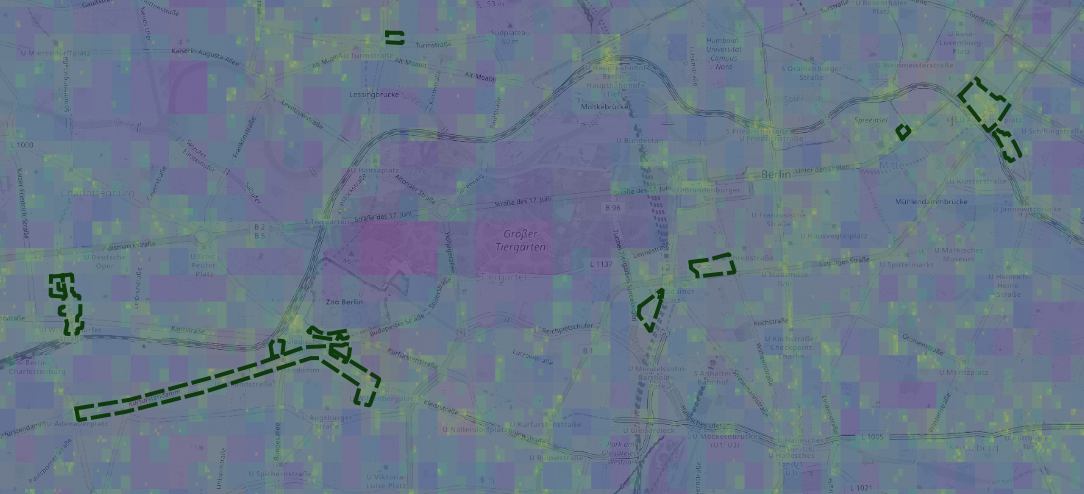retail areas and densities

Compared to mobile data we have a completely different ranking of locations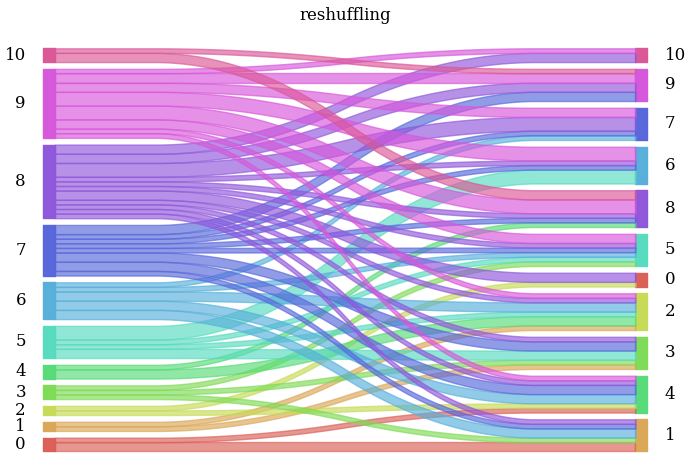re shuffling of ranking of retail areas__